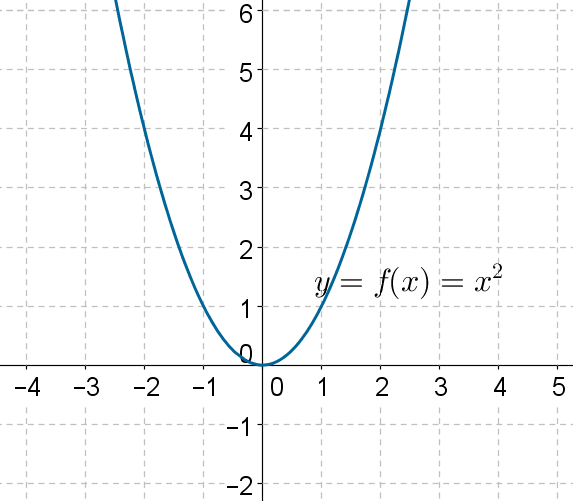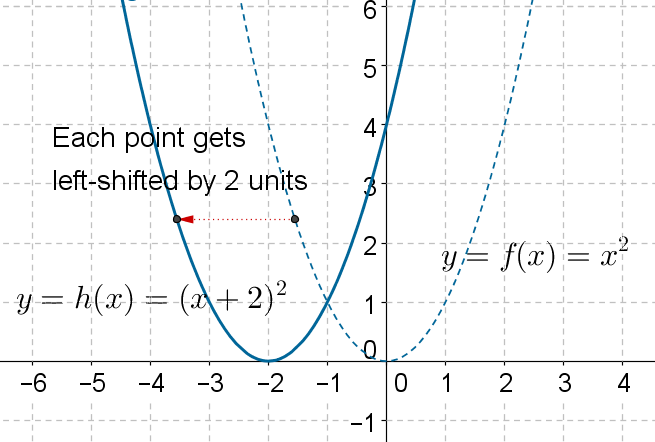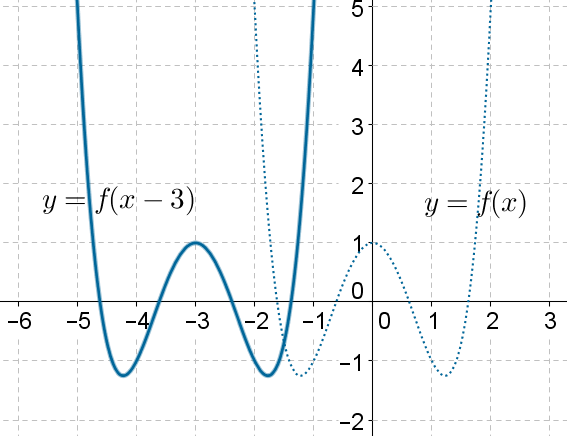# Horizontal Translations

Go back to  'Functions'

The following figure shows the graph of $$y = f\left( x \right) = {x^2}$$:Using this graph, can we plot the graph of the function $$g\left( x \right) ={\left( {x - 2} \right)^2}$$?

We make some example observations:

•  The zero of the original function f is at $$x= 0$$; the zero of the function g will be at $$x = 2$$. Thus, the zero will right-shift by 2 units.

• The function f attains the value of 1 for the values $$x = \pm 1$$; the function g will attain the same value of 1 for $$x = 1,3$$.Once again, relative to the original graph, these values have right-shifted by 2 units.

Clearly, any value which the function f attains at an input value of $$x ={x_0}$$ will be attained by g at an input value of $$x = {x_0} + 2$$. In other words, if $$\left( {{x_0},{y_0}} \right)$$ is a point on the graph of f, then $$\left( {{x_0} +2,{y_0}} \right)$$ will be a point on the graph of g. This indicates that the whole graph will get right shifted by 2 units:Similarly, the following figure shows how the graph of the function $$h\left( x\right) = {\left( {x + 2} \right)^2}$$ can be obtained from the graph of by left-shifting it by 2 units:In general, the graph of $$y = f\left( {x + k} \right)$$ can be obtained by horizontally shifting the graph of $$y =f\left( x \right)$$ by $$\left| k \right|$$units . The direction of the shift will depend upon the sign of k. If is positive, the shift will be leftward, else it will be rightward.

As another example, consider the following figure:The dotted curve corresponds to the graph of some arbitrary function, $$y = f\left(x \right)$$. The solid curve represents $$y= f\left( {x + 3} \right)$$. Note how each point on the original curve can be left-shifted by 3 units to obtain the new curve.

Functions
Functions
grade 10 | Questions Set 2
Functions
Functions
grade 10 | Questions Set 1